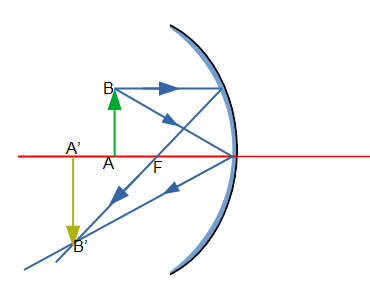# An object 0.600 cm tall is placed 16.5 cm to the left of the vertex of a concave mirror having a...

## Question:

An object 0.600 cm tall is placed 16.5 cm to the left of the vertex of a concave mirror having a radius of curvature of 22.0 cm.

a) Draw a principle-ray diagram showing the formation of the image.

b) Calculate the position, size, orientation (erect or inverted), and nature (real or virtual) of the image.

## Image formation by a Concave Mirror and Mirror Formula:

Image formation by Concave Mirror

• When the object lies beyond the center of curvature, the image always forms in between the center of curvature and the focal point (focus of the mirror).
• When the object lies between the focal point and the mirror, the image always forms on the opposite side of the mirror.

Mirror formula

Mirror formula is relation between the object distance, image distances and the focal length of the mirror, It is expressed by the equation;

{eq}\dfrac {1}{v}+\dfrac {1}{u} = \dfrac {1}{f}\\ {/eq}

where,

• {eq}v {/eq} is the image distance,
• {eq}u {/eq} is the object distance and
• {eq}f {/eq} is the focal length of the mirror.

Given

• Height of the object is {eq}h_o= 0.6\ cm {/eq}
• Distance of object from the mirror is {eq}u= -16.5\ cm {/eq}
• Radius of curvature of the mirror is {eq}R= -22 \ cm {/eq}

Part (a)

The ray diagram of the current situation is shown in the figure below.In the above figure,

• AB is the object and A'B' is the image of that object.

Part (b)

As we know that the radius of curvature is given by;

{eq}\begin{align} R &= 2f\\ -22\ cm& = 2f\\ \implies f&= -11\ cm\\ \end{align} {/eq}

From the Mirror formula,

{eq}\begin{align} \dfrac {1}{f}&=\dfrac{1}{v}+\dfrac {1}{u}\\ \implies \dfrac{1}{v}&= \dfrac{1}{f}-\dfrac{1}{u}\\ \implies \dfrac{1}{v}&= \dfrac{1}{-11\ cm}- \dfrac{1}{-16.5\ cm}\\ \implies v &= -33\ cm\\ \end{align} {/eq}

The magnification of the image is given by;

{eq}\begin{align} m &= -\dfrac {v}{u}\\ \implies \dfrac{1}{2}&=-\dfrac {-33\ cm}{-16.5\ cm}\\ \implies m &= -2\\ \end{align} {/eq}

Also the magnification of the image is given by;

{eq}\begin{align} m &= \dfrac {h_i}{h_o}\\ \implies -2 &=\dfrac {h_i}{0.6\ cm}\\ \implies h_i&= -1.2\ cm\\ \end{align} {/eq}

Thus, the image formed at a distance of {eq}33\ cm {/eq} in front of the mirror, its height is 1.2 cm, it is real in nature and it is inverted.